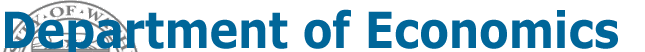Home
Syllabus
Homework
Notes
Announcements

# Production Function

Winter 2000

Last updated: January 25, 2000

Note: These notes are preliminary and incomplete and they are not guaranteed to be free of errors. Please let me know if you find typos or other errors.

## The Production Function

The aggregate production function for the economy is assumed to take the form

## Y = A·F(K, N)

where

• Y = real GDP
• A = index of overall productivity in the economy
• K = amount of capital input (measured in physical units or in \$ value) in the economy
• N = number of workers employed in the economy

### Assumptions concerning the shape of F(K, N)

• Marginal product of labor, MNP, is positive and diminishing ( MPN = DY/DN holding everything else fixed)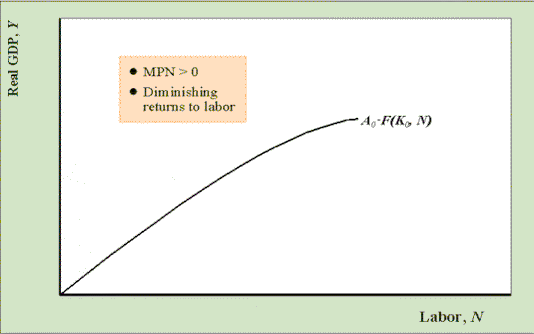The graph above shows the production function as a function of N holding A and K fixed.

• Marginal product of capital, MPK, is positive and diminishing (MPK = DY/DK holding everything else fixed)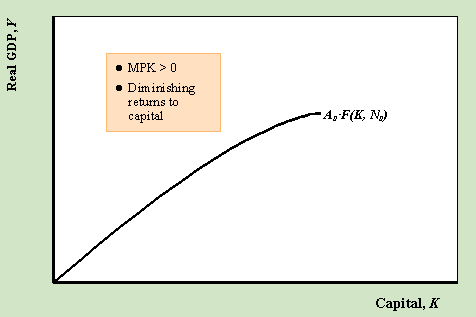The graph above shows the production function as a function of K holding A and N fixed.

#### Example: Cobb-Douglas constant returns to scale production function

Y = A·KaN1-a , 0 < a < 1

where

• a = share of income received by owners of capital
• 1 - a = share of income received by labor

Note: Constant returns to scale means that if we double all inputs to the production function then output exactly doubles.  To see that the Cobb-Douglas exhibits this property, note that

A·(2K)a(2N)1-a   = A·2a21-aKaN1-a   = 2A·KaN1-a  = 2Y

For US data, from the national income and product accounts, we know that labor's share of income is about 0.7 which implies that for the US 1-a = 0.7 and a = 0.3.

## A Medium-run Assumption

The production function shows how real GDP, Y, is determined over some fixed time period like a quarter or a year. Over this time period it is assumed that capital stock, K, and the level of overall productivity, A, is held fixed whereas it is assumed that the amount of labor can vary. That is, over the assumed time period, firms in the economy cannot easily alter the amount of capital they use (i.e. buildings, equipment etc.) but they can easily vary the amount of labor that they hire. Similarly, it is assumed that productivity does not change very quickly.  Given, these assumptions we can represent the production function as

Y = A0F(K0, N)

where the subscript "0" indicates that the level of productivity is fixed at the amount A0 and the level of capital is fixed at the amount K0. The unsubscripted variable, N, is allowed to vary.

## Moving Along vs. Shifting Curves

#### Moving along the production function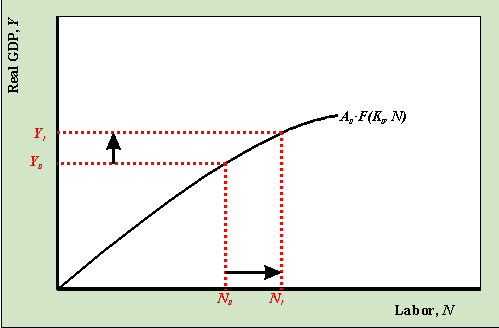As N increases from N0 to N1 output increases from Y0 to Y1 as we move along the production function.

#### Shifting the production function: An increase in productivity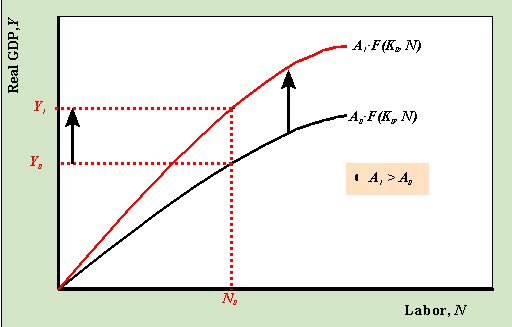When the index of productivity increases from A0 to A1, holding everything else fixed, the production function shifts up. Then for a given amount of labor, N0, the amount of output produced in the economy increases from Y0 to Y1.

#### Shifting the production function: An increase in the stock of capital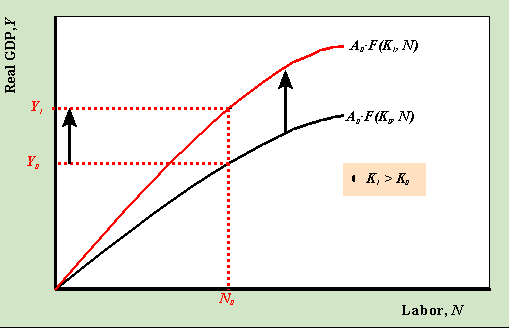When the capital stock increases from K0 to K1, holding everything else fixed, the production function shifts up. Then for a given amount of labor, N0, the amount of output produced in the economy increases from Y0 to Y1.